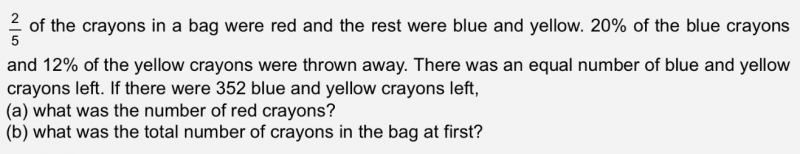# QuestionPlz help. No algebra needed.

Number of blue crayons at the end = 352/2 = 176
Number of blue crayons originally = 176/0.8 = 220
Number of yellow crayons at the end = 176
Number of yellow crayons originally = 176/0.88 = 200
Number of blue + yellow crayons originally = 220 + 200 = 420 (3/5 of the total crayons)
(a) Number of red crayons = 420/3 x2 = 280
(b) total number of crayons = 420/3 x5=700

0 Replies 1 Like

(a)                                  Red                      Blue                    Yellow

At first                           2u                                       3u

Thrown away                                            20%                       12%

Remaining                    2u                        80%                       88%

Remaining blue and yellow are equal and total = 352

=> Blue = 176    and    Yellow = 176

80% Blue —> 176   =>  100% Blue =  (176/80) x 100 = 220

88% Yellow —> 176 =>  100% Yellow = (176/88) x 100 = 200

Total Blue and Yellow = 220 + 200 = 420

3u –> 420  =>  2u = (420/3) x 2 = 280

Hence Red crayons is 280

(b) Total crayons at first = 280+420 =700

0 Replies 1 Like